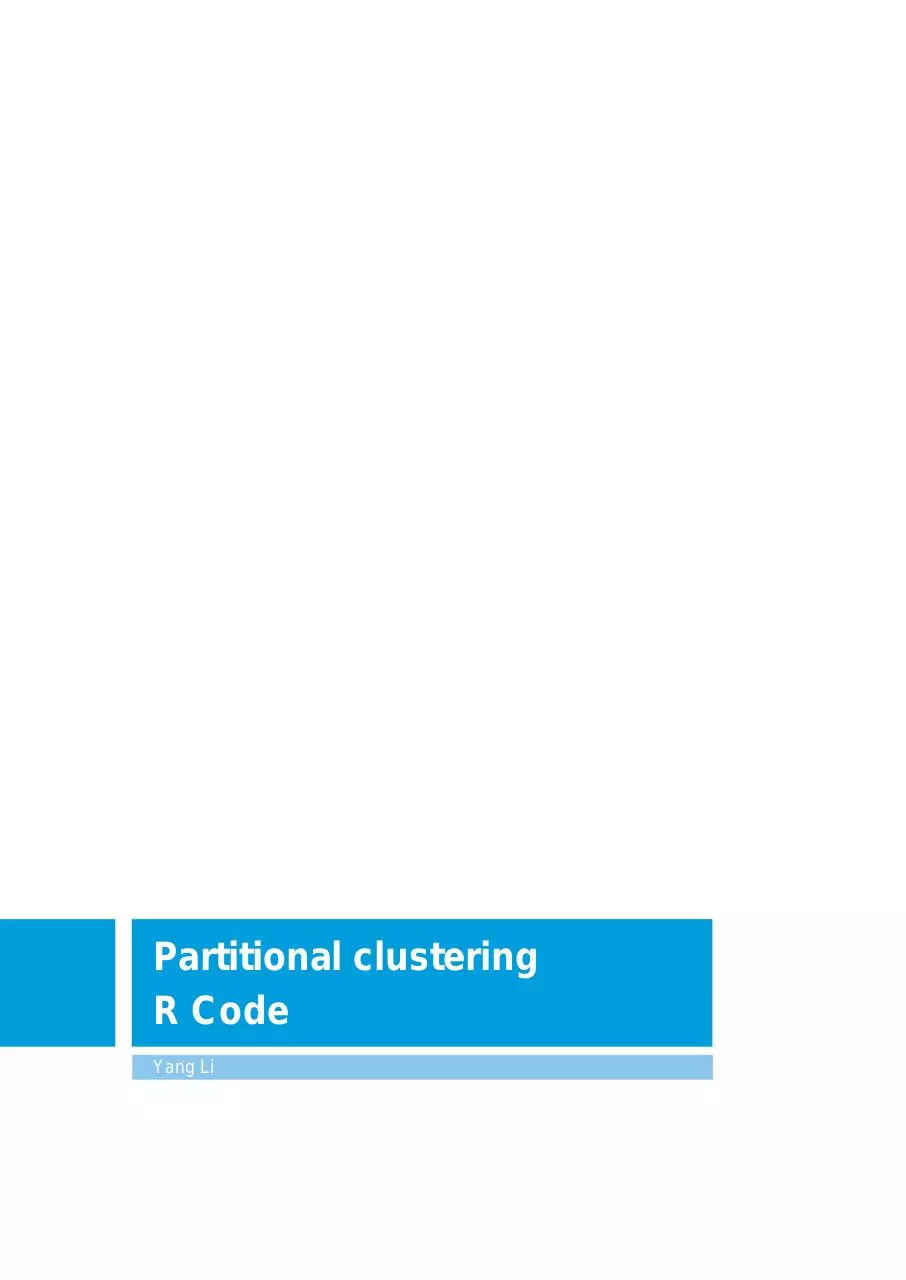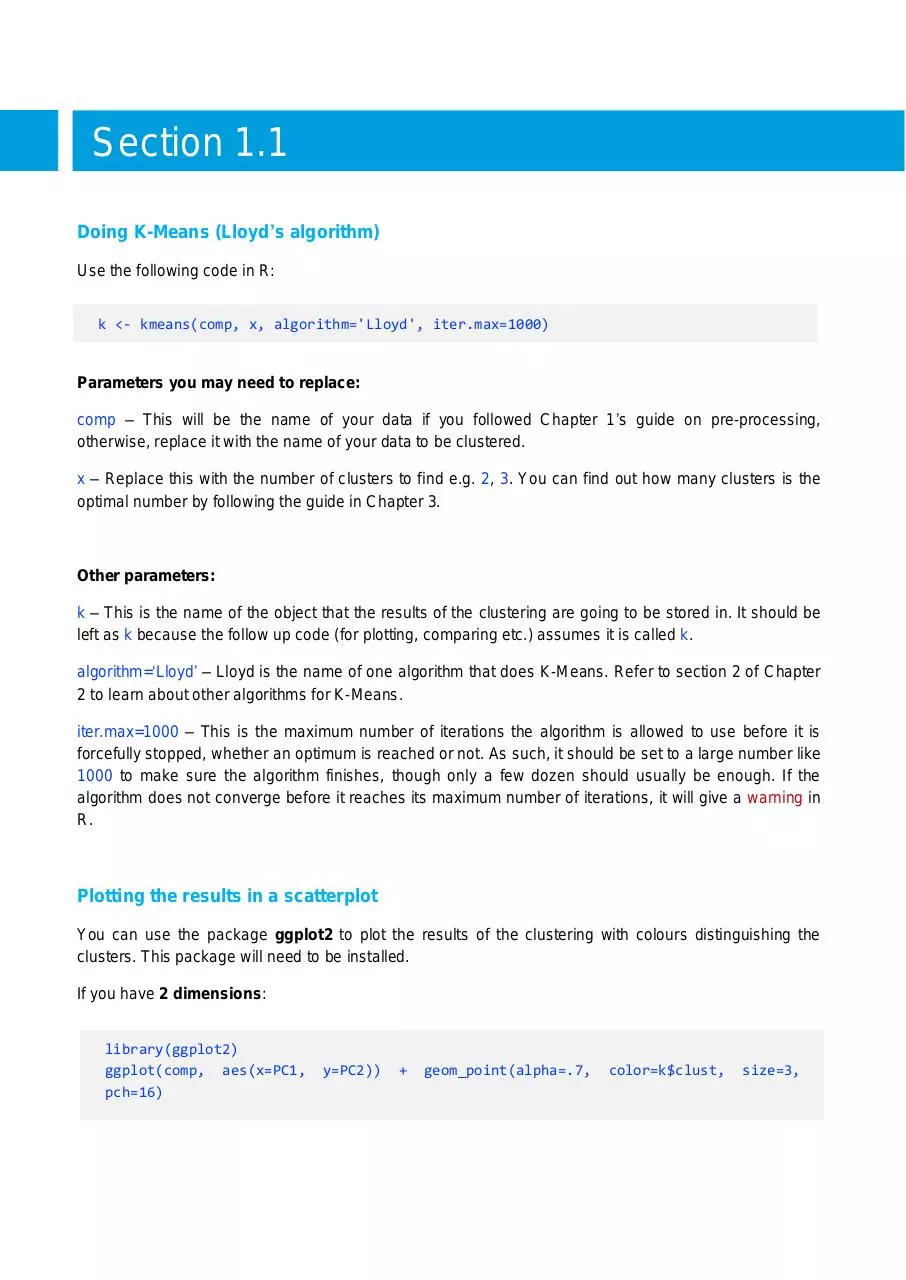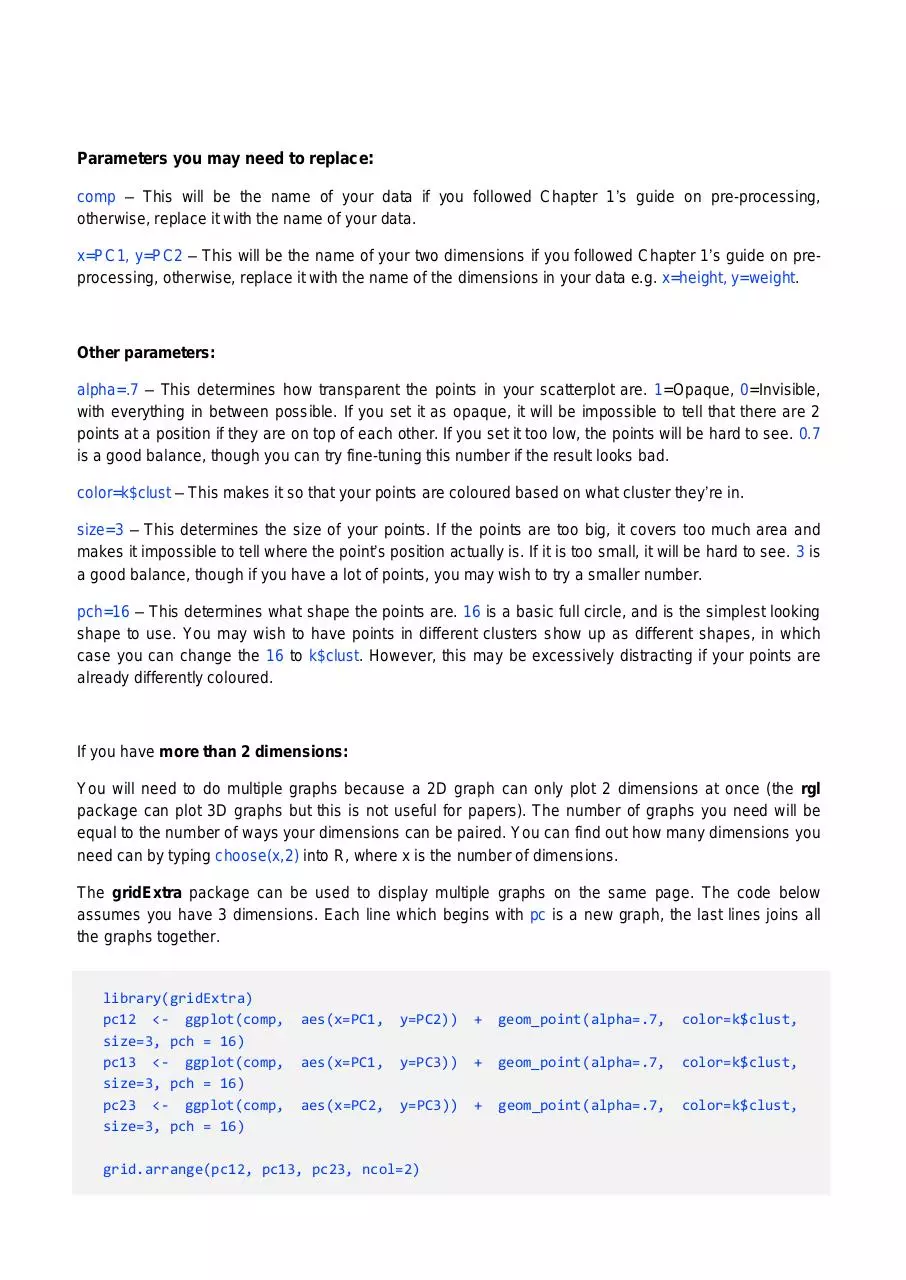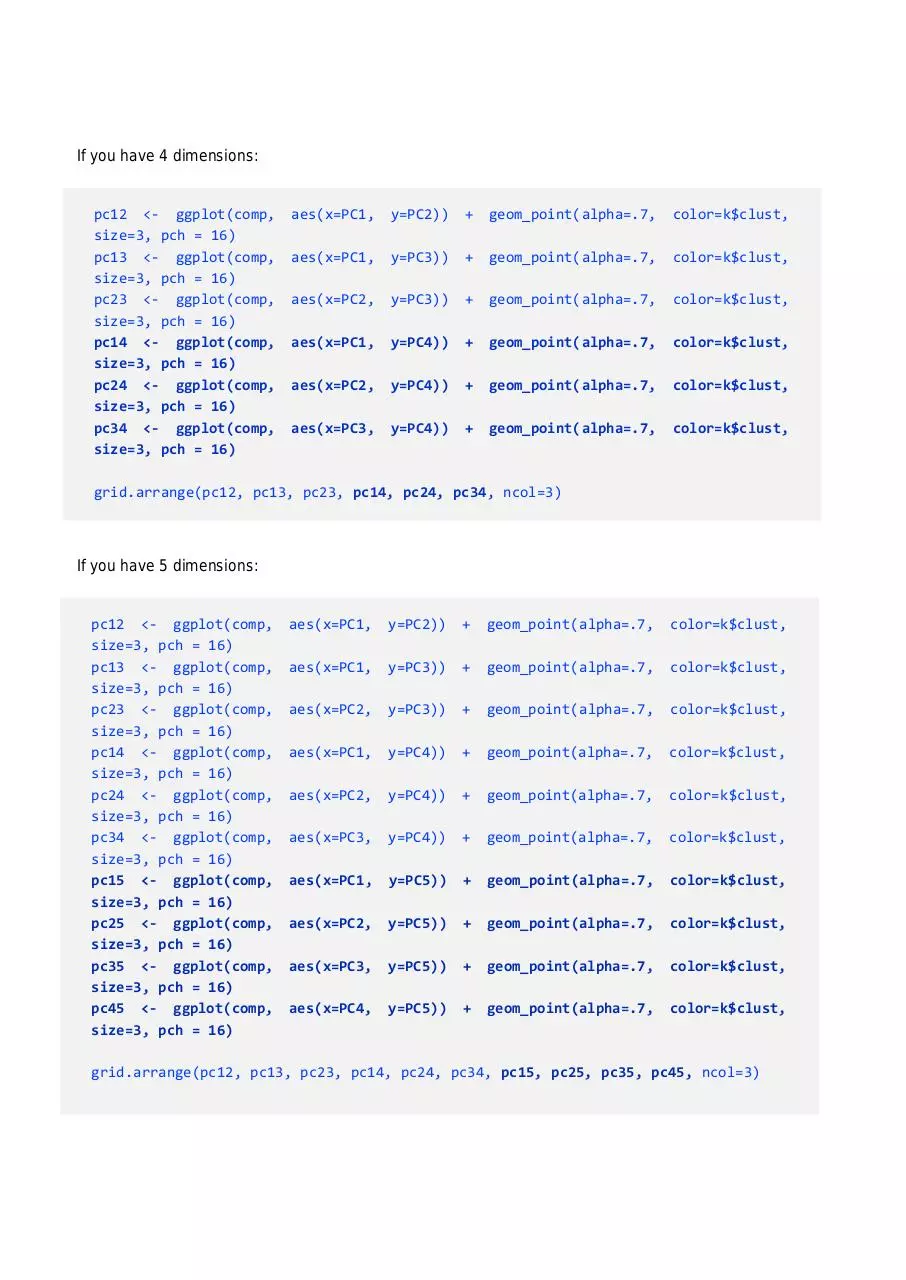# 2B R Code .pdf

### File information

Author: Yang Li

This PDF 1.5 document has been generated by MicrosoftÂ® Word 2013, and has been sent on pdf-archive.com on 25/01/2016 at 20:21, from IP address 222.154.x.x. The current document download page has been viewed 653 times.
File size: 872.6 KB (102 pages).
Privacy: public file

### Document preview

Partitional clustering
R Code
Yang Li

Contents

Section 1.1
Doing K-Means (Lloyd’s algorithm)
Use the following code in R:
k &lt;- kmeans(comp, x, algorithm='Lloyd', iter.max=1000)

Parameters you may need to replace:
comp – This will be the name of your data if you followed Chapter 1’s guide on pre-processing,
otherwise, replace it with the name of your data to be clustered.
x – Replace this with the number of clusters to find e.g. 2, 3. You can find out how many clusters is the
optimal number by following the guide in Chapter 3.

Other parameters:
k – This is the name of the object that the results of the clustering are going to be stored in. It should be
left as k because the follow up code (for plotting, comparing etc.) assumes it is called k.
algorithm=‘Lloyd’ – Lloyd is the name of one algorithm that does K-Means. Refer to section 2 of Chapter
2 to learn about other algorithms for K-Means.
iter.max=1000 – This is the maximum number of iterations the algorithm is allowed to use before it is
forcefully stopped, whether an optimum is reached or not. As such, it should be set to a large number like
1000 to make sure the algorithm finishes, though only a few dozen should usually be enough. If the
algorithm does not converge before it reaches its maximum number of iterations, it will give a warning in
R.

Plotting the results in a scatterplot
You can use the package ggplot2 to plot the results of the clustering with colours distinguishing the
clusters. This package will need to be installed.
If you have 2 dimensions:

library(ggplot2)
ggplot(comp, aes(x=PC1,
pch=16)

y=PC2))

+

geom_point(alpha=.7,

color=k\$clust,

size=3,

Parameters you may need to replace:
comp – This will be the name of your data if you followed Chapter 1’s guide on pre-processing,
otherwise, replace it with the name of your data.
x=PC1, y=PC2 – This will be the name of your two dimensions if you followed Chapter 1’s guide on preprocessing, otherwise, replace it with the name of the dimensions in your data e.g. x=height, y=weight.

Other parameters:
alpha=.7 – This determines how transparent the points in your scatterplot are. 1=Opaque, 0=Invisible,
with everything in between possible. If you set it as opaque, it will be impossible to tell that there are 2
points at a position if they are on top of each other. If you set it too low, the points will be hard to see. 0.7
is a good balance, though you can try fine-tuning this number if the result looks bad.
color=k\$clust – This makes it so that your points are coloured based on what cluster they’re in.
size=3 – This determines the size of your points. If the points are too big, it covers too much area and
makes it impossible to tell where the point’s position actually is. If it is too small, it will be hard to see. 3 is
a good balance, though if you have a lot of points, you may wish to try a smaller number.
pch=16 – This determines what shape the points are. 16 is a basic full circle, and is the simplest looking
shape to use. You may wish to have points in different clusters show up as different shapes, in which
case you can change the 16 to k\$clust. However, this may be excessively distracting if your points are

If you have more than 2 dimensions:
You will need to do multiple graphs because a 2D graph can only plot 2 dimensions at once (the rgl
package can plot 3D graphs but this is not useful for papers). The number of graphs you need will be
equal to the number of ways your dimensions can be paired. You can find out how many dimensions you
need can by typing choose(x,2) into R, where x is the number of dimensions.
The gridExtra package can be used to display multiple graphs on the same page. The code below
assumes you have 3 dimensions. Each line which begins with pc is a new graph, the last lines joins all
the graphs together.

library(gridExtra)
pc12 &lt;- ggplot(comp,
size=3, pch = 16)
pc13 &lt;- ggplot(comp,
size=3, pch = 16)
pc23 &lt;- ggplot(comp,
size=3, pch = 16)

aes(x=PC1,

y=PC2))

+

geom_point(alpha=.7,

color=k\$clust,

aes(x=PC1,

y=PC3))

+

geom_point(alpha=.7,

color=k\$clust,

aes(x=PC2,

y=PC3))

+

geom_point(alpha=.7,

color=k\$clust,

grid.arrange(pc12, pc13, pc23, ncol=2)

If you have 4 dimensions:

pc12 &lt;- ggplot(comp,
size=3, pch = 16)
pc13 &lt;- ggplot(comp,
size=3, pch = 16)
pc23 &lt;- ggplot(comp,
size=3, pch = 16)
pc14 &lt;- ggplot(comp,
size=3, pch = 16)
pc24 &lt;- ggplot(comp,
size=3, pch = 16)
pc34 &lt;- ggplot(comp,
size=3, pch = 16)

aes(x=PC1,

y=PC2))

+

geom_point(alpha=.7,

color=k\$clust,

aes(x=PC1,

y=PC3))

+

geom_point(alpha=.7,

color=k\$clust,

aes(x=PC2,

y=PC3))

+

geom_point(alpha=.7,

color=k\$clust,

aes(x=PC1,

y=PC4))

+

geom_point(alpha=.7,

color=k\$clust,

aes(x=PC2,

y=PC4))

+

geom_point(alpha=.7,

color=k\$clust,

aes(x=PC3,

y=PC4))

+

geom_point(alpha=.7,

color=k\$clust,

grid.arrange(pc12, pc13, pc23, pc14, pc24, pc34, ncol=3)

If you have 5 dimensions:

pc12 &lt;- ggplot(comp,
size=3, pch = 16)
pc13 &lt;- ggplot(comp,
size=3, pch = 16)
pc23 &lt;- ggplot(comp,
size=3, pch = 16)
pc14 &lt;- ggplot(comp,
size=3, pch = 16)
pc24 &lt;- ggplot(comp,
size=3, pch = 16)
pc34 &lt;- ggplot(comp,
size=3, pch = 16)
pc15 &lt;- ggplot(comp,
size=3, pch = 16)
pc25 &lt;- ggplot(comp,
size=3, pch = 16)
pc35 &lt;- ggplot(comp,
size=3, pch = 16)
pc45 &lt;- ggplot(comp,
size=3, pch = 16)

aes(x=PC1,

y=PC2))

+

geom_point(alpha=.7,

color=k\$clust,

aes(x=PC1,

y=PC3))

+

geom_point(alpha=.7,

color=k\$clust,

aes(x=PC2,

y=PC3))

+

geom_point(alpha=.7,

color=k\$clust,

aes(x=PC1,

y=PC4))

+

geom_point(alpha=.7,

color=k\$clust,

aes(x=PC2,

y=PC4))

+

geom_point(alpha=.7,

color=k\$clust,

aes(x=PC3,

y=PC4))

+

geom_point(alpha=.7,

color=k\$clust,

aes(x=PC1,

y=PC5))

+

geom_point(alpha=.7,

color=k\$clust,

aes(x=PC2,

y=PC5))

+

geom_point(alpha=.7,

color=k\$clust,

aes(x=PC3,

y=PC5))

+

geom_point(alpha=.7,

color=k\$clust,

aes(x=PC4,

y=PC5))

+

geom_point(alpha=.7,

color=k\$clust,

grid.arrange(pc12, pc13, pc23, pc14, pc24, pc34, pc15, pc25, pc35, pc45, ncol=3)

If you need to add even more dimensions, study what was added to the code (new parts in bold) and
follow the pattern. Do not forget to add more objects to the grid.arrange list in the last line.

Parameters you may need to replace:
comp – This will be the name of your data if you followed Chapter 1’s guide on pre-processing,
otherwise, replace it with the name of your data to be clustered.
x=PC1, y=PC2 – This is present in a slightly altered form in each line. It should be the name of the two
dimensions to be plotted for that graph. Replace it with the name of the dimensions in your data e.g.
x=height, y=weight for one line, then x=height, y=age for the next line etc.

Other parameters:
alpha=.7 – This determines how transparent the points in your scatterplot are. 1=Opaque, 0=Invisible,
with everything in between possible. If you set it as opaque, it will be impossible to tell that there are 2
points at a position if they are on top of each other. If you set it too low, the points will be hard to see. 0.7
is a good balance, though you may try fine-tuning this number if the result looks bad.
color=k\$clust – This makes it so that your points are coloured based on what cluster they’re in.
size=3 – This determines the size of your points. If the points are too big, it covers too much area and
makes it impossible to tell where the point’s position actually is. If it is too small, it will be hard to see. 3 is
a good balance, though if you have a lot of points, you may wish to try a smaller number.
pch=16 – This determines what shape the points are. 16 is a basic full circle, and is the simplest looking
shape to use. You may wish to have points in different clusters show up as different shapes, in which
case you can change the 16 to k\$clust. However, this may be excessively distracting if your points are
ncol=3 – This is the number of columns to arrange the graphs in. If you have more graphs you will need
to increase this number, though too many graphs do not fit well on one page. You can also use nrow=3
to set the number of rows.

Seeing what’s in each cluster
You can list the objects in each cluster with the following code:
clust &lt;- names(sort(table(k\$clust)))
clustnumb &lt;- length(clust)
for(i in 1:clustnumb){
print(c("Cluster number:", clust[i]))
print(row.names(comp[k\$clust==clust[i],]))
}
sort(table(k\$clust))

It lists the clusters by order of size. Because of this, you must be careful when comparing two different
clustering algorithms on the same data. The order of clusters may not be the same between the 2
algorithms.

Parameters you may need to replace:
comp – This will be the name of your data if you followed Chapter 1’s guide on pre-processing,
otherwise, replace it with the name of your data to be clustered.

Comparing clusters by each original variable
You can see how the individuals in your cluster compare by plotting boxplots for all of your original
variables (before doing a PCA). The package ggplot2 will be used again.
First, you need to add a new column to your data which can be used to separate the individuals into
clusters for the boxplots.

NAMEOFDATA\$Cluster &lt;- k\$cluster

Parameters you may need to replace:
NAMEOFDATA – Replace this with the name of your original data (before all pre-processing steps
except data cleaning). Keep the \$Cluster part on the end.
Now you can compare the clusters across one variable using the following code:

qplot(factor(Cluster),
VARIABLE,
geom
=
"boxplot",
xlab='Cluster', ylab='VARIABLE') + geom_boxplot(aes(fill
theme(legend.position="NULL")

data
=
NAMEOFDATA,
= factor(Cluster))) +

Parameters you may need to replace:
NAMEOFDATA – Replace this with the name of your original data (before all pre-processing steps
except data cleaning).
VARIABLE – Replace this with the name of the variable. This is the column heading from your original
data you would like to compare the clusters by e.g. height. Note that you need to replace it in 2 places in
the code.

If you want to display multiple boxplots on one page, you can use gridExtra again.
box1 &lt;- qplot(factor(Cluster), VARIABLE1, geom = "boxplot", data = NAMEOFDATA,
xlab='Cluster', ylab='VARIABLE1') + geom_boxplot(aes(fill = factor(Cluster))) +
theme(legend.position="NULL")
box2 &lt;- qplot(factor(Cluster), VARIABLE2, geom = "boxplot", data = NAMEOFDATA,
xlab='Cluster', ylab='VARIABLE2') + geom_boxplot(aes(fill = factor(Cluster))) +
theme(legend.position="NULL")
box3 &lt;- qplot(factor(Cluster), VARIABLE3, geom = "boxplot", data = NAMEOFDATA,
xlab='Cluster', ylab='VARIABLE3') + geom_boxplot(aes(fill = factor(Cluster))) +
theme(legend.position="NULL")
grid.arrange(box1, box2, box3, ncol=2)

Parameters you may need to replace:
NAMEOFDATA – Replace this with the name of your original data (before all pre-processing steps
except data cleaning).
VARIABLE1, VARIABLE2, VARIABLE3 etc. – Replace these with the names of the variables i.e. column
headings from your original data you would like to compare the clusters by. Note that for each one, there
are 2 places in the code to replace them.
Adding extra graphs is easy, just don’t forget to add box4, box5 etc. to the grid.arrange list in the last line.

Section 2.1
Doing K-Means (MacQueen’s algorithm)
Use the following code in R:
k &lt;- kmeans(comp, x, algorithm='MacQueen', iter.max=1000)

Parameters you may need to replace:
comp – This will be the name of your data if you followed Chapter 1’s guide on pre-processing,
otherwise, replace it with the name of your data to be clustered.
x – Replace this with the number of clusters to find e.g. 2, 3. You can find out how many clusters is the
optimal number by following the guide in Chapter 3.

Other parameters:
k – This is the name of the object that the results of the clustering are going to be stored in. It should be
left as k because the follow up code (for plotting, comparing etc.) assumes it is called k.
algorithm=‘MacQueen’ – MacQueen is the name of one algorithm that does K-Means. Refer to section 2
of Chapter 2 to learn about other algorithms for K-Means.
iter.max=1000 – This is the maximum number of iterations the algorithm is allowed to use before it is
forcefully stopped, whether an optimum is reached or not. As such, it should be set to a large number like
1000 to make sure the algorithm finishes, though only a few dozen should usually be enough. If the
algorithm does not converge before it reaches its maximum number of iterations, it will give a warning in
R.

Plotting the results in a scatterplot
You can use the package ggplot2 to plot the results of the clustering with colours distinguishing the
clusters. This package will need to be installed.
If you have 2 dimensions:

library(ggplot2)
ggplot(comp, aes(x=PC1,
pch=16)

y=PC2))

+

geom_point(alpha=.7,

color=k\$clust,

size=3,2B - R Code.pdf (PDF, 872.6 KB)

### Share on social networks

#### HTML Code

Copy the following HTML code to share your document on a Website or Blog